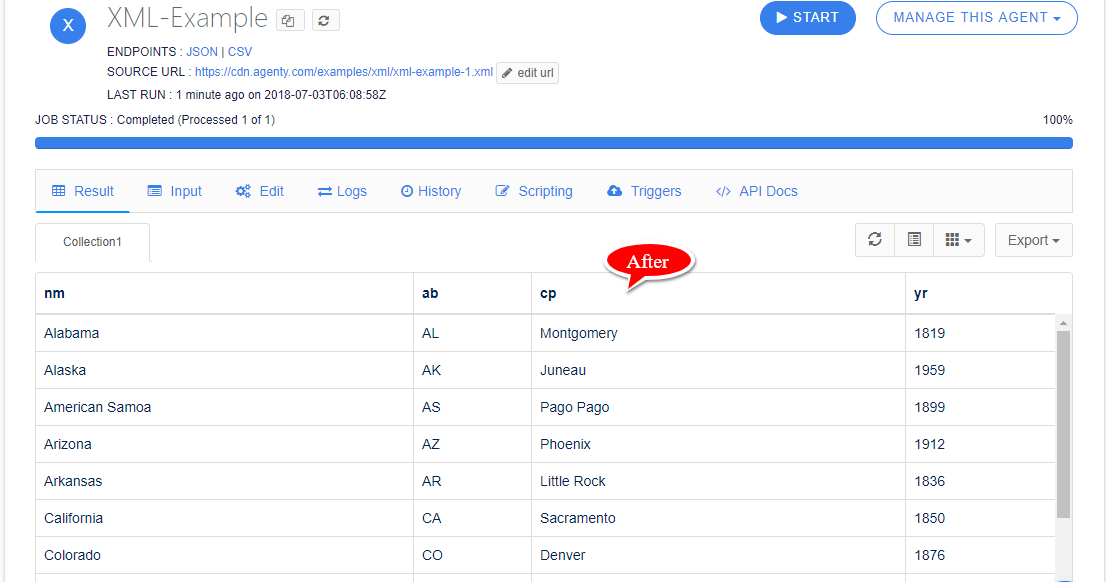# XML Scraping

In this tutorial, we will learn how to extract data from XML using scraping agent. An XML is a metalanguage, which allows users to define their own customized markup languages, especially in order to display documents on the Internet. So, many websites or API uses the XML markup to display the content, sitemap or their data feeds online and it's crucial to have the ability to scrape structured content from XML pages using a scraping agent.

## XML Example

I have this URL https://cdn.agenty.com/examples/xml/xml-example-1.xml where the content is displaying as XML markup as given below:

``````<?xml version="1.0" encoding="utf-8"?>
<states>
<s nm="Alabama" ab="AL" cp="Montgomery" yr="1819" />
<s nm="Alaska" ab="AK" cp="Juneau" yr="1959" />
<s nm="American Samoa" ab="AS" cp="Pago Pago" yr="1899" />
<s nm="Arizona" ab="AZ" cp="Phoenix" yr="1912" />
<s nm="Arkansas" ab="AR" cp="Little Rock" yr="1836" />
<s nm="California" ab="CA" cp="Sacramento" yr="1850" />
<s nm="Colorado" ab="CO" cp="Denver" yr="1876" />
<s nm="Connecticut" ab="CT" cp="Hartford" yr="1788" />
<s nm="Delaware" ab="DE" cp="Dover" yr="1787" />
<s nm="Florida" ab="FL" cp="Tallahassee" yr="1845" />
<s nm="Georgia" ab="GA" cp="Atlanta" yr="1788" />
<s nm="Guam" ab="GU" cp="Hagåtña Dededo" yr="1898" />
<s nm="Hawaii" ab="HI" cp="Honolulu" yr="1959" />
<s nm="Idaho" ab="ID" cp="Boise" yr="1890" />
<s nm="Illinois" ab="IL" cp="Springfield" yr="1818" />
<s nm="Indiana" ab="IN" cp="Indianapolis" yr="1816" />
<s nm="Iowa" ab="IA" cp="Des Moines" yr="1846" />
<s nm="Kansas" ab="KS" cp="Topeka" yr="1861" />
<s nm="Kentucky" ab="KY" cp="Frankfort" yr="1792" />
<s nm="Louisiana" ab="LA" cp="Baton Rouge" yr="1812" />
<s nm="Maine" ab="ME" cp="Augusta" yr="1820" />
<s nm="Maryland" ab="MD" cp="Annapolis" yr="1788" />
<s nm="Massachusetts" ab="MA" cp="Boston" yr="1788" />
<s nm="Michigan" ab="MI" cp="Lansing" yr="1837" />
<s nm="Minnesota" ab="MN" cp="Saint Paul" yr="1858" />
<s nm="Mississippi" ab="MS" cp="Jackson" yr="1817" />
<s nm="Missouri" ab="MO" cp="Jefferson City" yr="1821" />
<s nm="Montana" ab="MT" cp="Helena" yr="1889" />
<s nm="Nebraska" ab="NE" cp="Lincoln" yr="1867" />
<s nm="Nevada" ab="NV" cp="Carson City" yr="1864" />
<s nm="New Hampshire" ab="NH" cp="Concord" yr="1788" />
<s nm="New Jersey" ab="NJ" cp="Trenton" yr="1787" />
<s nm="New Mexico" ab="NM" cp="Santa Fe" yr="1912" />
<s nm="New York" ab="NY" cp="Albany" yr="1788" />
<s nm="North Carolina" ab="NC" cp="Raleigh" yr="1789" />
<s nm="North Dakota" ab="ND" cp="Bismarck" yr="1889" />
<s nm="Northern Mariana Islands" ab="MP" cp="Saipan" yr="1947" />
<s nm="Ohio" ab="OH" cp="Columbus" yr="1803" />
<s nm="Oklahoma" ab="OK" cp="Oklahoma City" yr="1907" />
<s nm="Oregon" ab="OR" cp="Salem" yr="1859" />
<s nm="Pennsylvania" ab="PA" cp="Harrisburg" yr="1787" />
<s nm="Puerto Rico" ab="PR" cp="San Juan" yr="1898" />
<s nm="Rhode Island" ab="RI" cp="Providence" yr="1790" />
<s nm="South Carolina" ab="SC" cp="Columbia" yr="1788" />
<s nm="South Dakota" ab="SD" cp="Pierre" yr="1889" />
<s nm="Tennessee" ab="TN" cp="Nashville" yr="1796" />
<s nm="Texas" ab="TX" cp="Austin" yr="1845" />
<s nm="U.S. Virgin Islands" ab="VI" cp="Charlotte Amalie" yr="1917" />
<s nm="Utah" ab="UT" cp="Salt Lake City" yr="1896" />
<s nm="Vermont" ab="VT" cp="Montpelier" yr="1791" />
<s nm="Virginia" ab="VA" cp="Richmond" yr="1788" />
<s nm="Washington" ab="WA" cp="Olympia" yr="1889" />
<s nm="Washington D.C." ab="DC" cp="" yr="" />
<s nm="West Virginia" ab="WV" cp="Charleston" yr="1863" />
<s nm="Wisconsin" ab="WI" cp="Madison" yr="1848" />
<s nm="Wyoming" ab="WY" cp="Cheyenne" yr="1890" />
</states>
``````

Now, if you look on the above content, It's not an HTML page, where we can use our Chrome extension to generate CSS selectors automatically. So, we need to create our agent manually and then edit that in Agenty, to add, update the fields and URL...so, let's create a dummy agent

## Create Agent

1. Go to agents.
2. Click on `New Agent`
3. Get any of the example agent available in `Sample agent` section. (Because we are going to edit the url, selector, fields...so we can use any demo agent, to create one and then edit per our need).

## Regex

We will be using REGEX to extract the fields from the XML page. So, use any online REGEX tester for create our expression, I am going to use rubular.com in this example, to demonstrate the expression and result. I have created a permanent link as well if you want to hands on : http://rubular.com/r/Jh1iGMPBR5.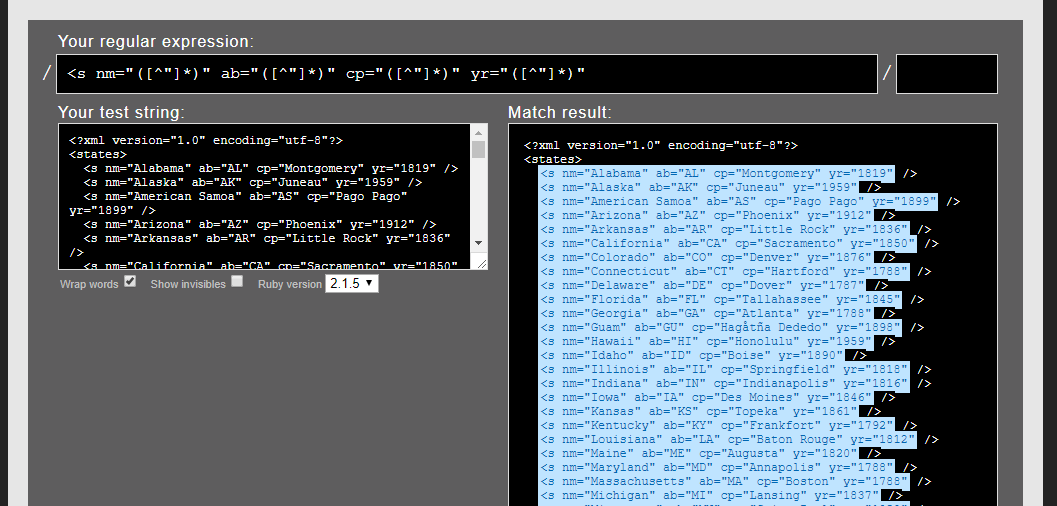So, my REGEX expression is given below, to try for all 4 fields with different group name or number.

<s nm="([^"]*)" ab="([^"]*)" cp="([^"]*)" yr="([^"]*)"

## Matching Groups Preview

If you see the screenshot given below, the expression I used has 4 matching result in each XML `s` tag, which are given default names as 1,2,3,4 in the group. So, we will be using this expression with group number to extract our desired result in field we need it.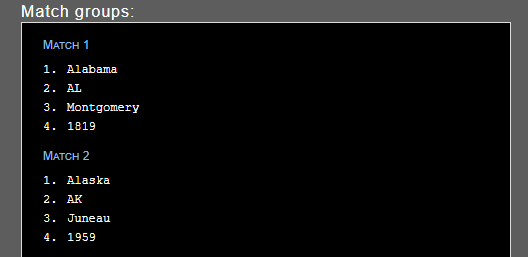Now, we need to edit the Scraping agent and then add the fields using the REGEX expression and group number as per last step.

1. Edit the scraping agent by clicking on the `Edit` tab,

2. Add a new field, name it nm,

3. Now select the Type as 'REGEX' and paste your expression in Regex Pattern and the Group index in Group Index for the first field,

4. Add one more field, and name it ab, Type as 'REGEX' and the Regex Pattern with 'Group Index' given in below screenshot.

Note:- I changed the Group index as "2" here because if you look on the result preview on "Matching group preview" section the ab value is on second position.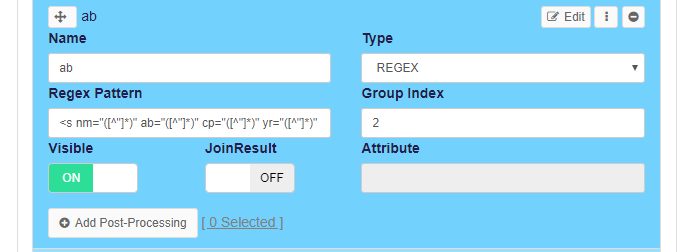6. And then, similarly for 3rd field by just changing the group index number and name of field

Note:- Two fields can't have the same name.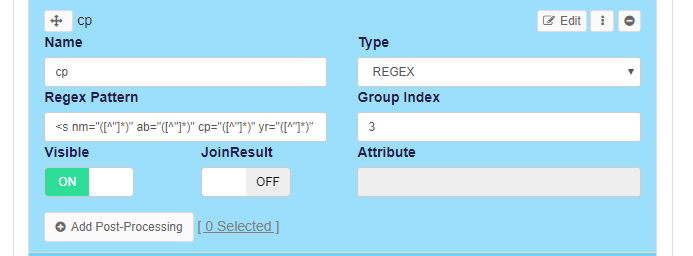7. Finally, the 4th field to extract the year value from yr attribute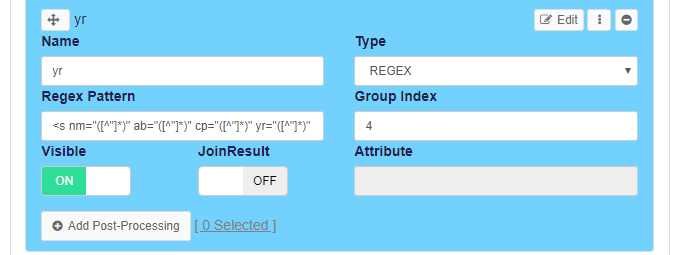8. Now `Save` the scraping agent configuration,

1. And, re-run your agent to refresh the result, as per the change in agent configuration.

## Execution

Once the job is completed, we can see the scraping agent result in structured format, by going to `Result` tab, and can add any number of URLs with similar structure of XML to scrape the XML pages or to run automatically using Agenty advance features or API to automate the XML scraping.